# SAT II Math II : Expanding Expressions and FOIL

## Example Questions

### Example Question #7 : Monomials

Expand the expression by multiplying the terms.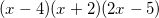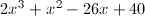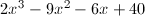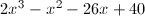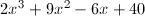Explanation:When multiplying, the order in which you multiply does not matter. Let's start with the first two monomials.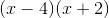Use FOIL to expand.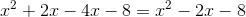Now we need to multiply the third monomial.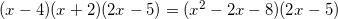Similar to FOIL, we need to multiply each combination of terms.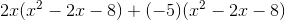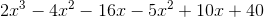Combine like terms.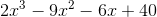### Example Question #1 : How To Multiply Trinomials

Multiply the expressions: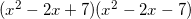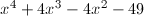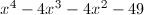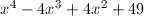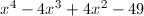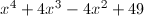Explanation:

You can look at this as the sum of two expressions multiplied by the difference of the same two expressions. Use the pattern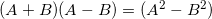,

where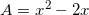and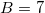.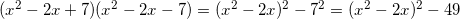To find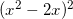, you use the formula for perfect squares: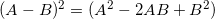,

where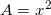and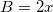.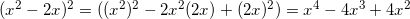Substituting above, the final answer is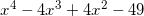.

### Example Question #1 : Expanding Expressions And Foil

Which of the following values ofwould make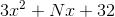a prime polynomial?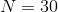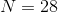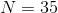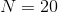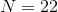Explanation:

A polynomial of the form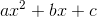whose terms do not have a common factor, such as this, can be factored by rewriting it as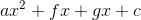such that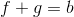and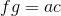; the grouping method can be used on this new polynomial.

Therefore, forto be factorable,must be the sum of the two integers of a factor pair of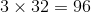. We are looking for a value ofthat is not a sum of two such factors.

The factor pairs of 96, along with their sums, are:

1 and 96 - sum 97

2 and 48 - sum 50

3 and 32 - sum 35

4 and 24 - sum 28

6 and 16 - sum 22

8 and 12 - sum 20

Of the given choices, only 30 does not appear among these sums; it is the correct choice.

### Example Question #1 : Expanding Expressions And Foil

How many of the following are prime factors of the polynomial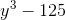?

(A)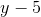(B)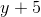(C)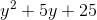(D)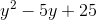One

Three

None

Four

Two

Two

Explanation: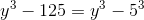making this polynomial the difference of two cubes.

As such,can be factored using the pattern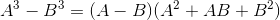so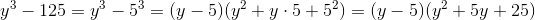(A) and (C) are both factors, but not (B) or (D), so the correct response is two.